# Calculating Sum of all Elements in an Array using C++

## Calculating Sum of all Elements in an Array using C++:

In this article, I am going to discuss the program for Calculating the sum of all elements in an array using C++ Language with examples. Please read our previous articles, where we discussed Foreach Loop in C++ with Examples.

##### Calculating the sum of all elements in an array using C++:

Let us write the program for finding the sum of all the elements in an array. Let us take an array of size ‘5’,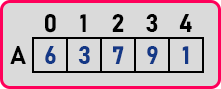We have an array of size of ‘7’ and indices start from 0 to 4. We want to add all these elements. So, this type of logic we have already seen by adding the numbers one by one i.e. sum of first n natural numbers. So, for that, we were taking the sum variable.

sum = 0;

So, we have taken ‘sum’, and initially, ‘sum’ is zero then, we have to add all the elements so that we can go through all those elements one by one. This will take us to all elements. So if we say A[i] then A is ‘6’, A is ‘3’, and so on. Now we want the sum.

##### Calculating the sum of all elements in an array using C++:

So, in that sum: 0 + 6 is ‘6’.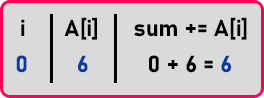Now that 6 + 3 is ‘9’.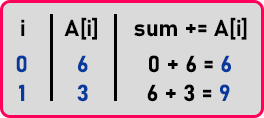And that 9 + 7 is ‘16’.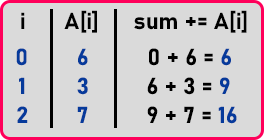Now 16 + 9 is ‘25’. And that 25 + 1 is ‘26’.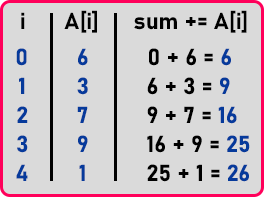So, in this way, we will calculate the sum of this array. So, this procedure you already know. Now let us write a program for it.

##### Program to Calculate the sum of elements of an array in C++:
```#include <iostream>
#include <conio.h>
using namespace std;

int main(){
int n, sum = 0;

cout << "Enter size of the array: ";
cin >> n;

int A[n];

cout << "Enter elements of the array:\n";
for(int i = 0; i < n; i++){
cin >> A[i];
sum += A[i];
}

cout << "Sum of the array: " << sum << endl;
getch();
}
```
###### Output: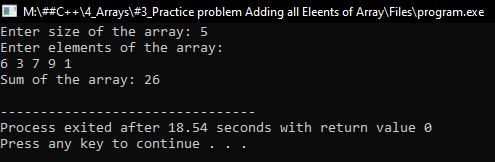So, this is a simple program to calculate the sum of all elements in an array using C++ Language.